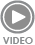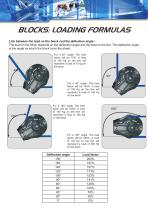{{requestButtons}}

## Catalog excerptsBLOCKS: LOADING FORMULAS Link between the load on the block and the deflection angle: The load on the block depends on the deflection angle and the load on the line. The deflection angle is the angle by which the block turns the sheet. For a 45° angle: The load factor will be 75%. A load of 100 Kg on the line will represent a load of 75 Kg on the block. 45° 90° For a 90° angle: The load factor will be 140%. A load of 100 Kg on the line will represent a load of 140 Kg on the block. For a 120° angle: The load factor will be 180%. A load of 100 Kg on the line will represent a load of 180 Kg on the block. For a 180° angle: The load factor will be 200%. A load of 100 Kg on the line will represent a load of 200 Kg on the block.

Open the catalog to page 1